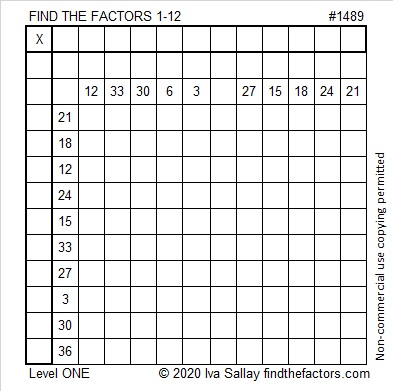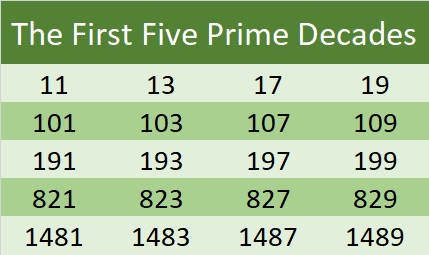# 1489 and Level 1

### Today’s Puzzle:

You can use the 20 clues in this puzzle to find the order that the factors from 1 to 12 must take. After you find those factors you can multiply each pair of factors together to complete the entire multiplication table.### Factors of 1489:

• 1489 is a prime number.
• Prime factorization: 1489 is prime.
• 1489 has no exponents greater than 1 in its prime factorization, so √1489 cannot be simplified.
• The exponent in the prime factorization is 1. Adding one to that exponent we get (1 + 1) = 2. Therefore 1489 has exactly 2 factors.
• The factors of 1489 are outlined with their factor pair partners in the graphic below.How do we know that 1489 is a prime number? If 1489 were not a prime number, then it would be divisible by at least one prime number less than or equal to √1489. Since 1489 cannot be divided evenly by 2, 3, 5, 7, 11, 13, 17, 19, 23, 29, 31, or 37, we know that 1489 is a prime number.

### Other Facts about the Number 1489:

1489 is the sum of two squares:
33² + 20² = 1489.

1489 is the hypotenuse of a Pythagorean triple:
689-1320-1489 calculated from 33² – 20², 2(33)(20), 33² + 20².

Here’s another way we know that 1489 is a prime number: Since its last two digits divided by 4 leave a remainder of 1, and 33² + 20² = 1489 with 33 and 20 having no common prime factors, 1489 will be prime unless it is divisible by a prime number Pythagorean triple hypotenuse less than or equal to √1489. Since 1489 is not divisible by 5, 13, 17, 29, or 37, we know that 1489 is a prime number.

1489 is the last prime number in the fifth prime decade:1489 is a centered triangular number because (3(31²) + 3(31) + 2)/2 = 1489.